# Lines, Line Segments and Rays

Lines, Line Segments and Rays

## What is a line?

A line is a set of points that extends in two opposite directions. Both ends of a line can be extended indefinitely. A line has no endpoints.

## What is a line segment?

A line segment is a part of a straight line that connects two points. It is the shortest path between the two points. It has a definite length, that is its length can be measured.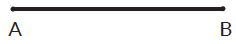## What is a ray?

A ray is a part of the line. It has a start point but no definite end point, and thus difficult to measure. It goes indefinitely in one direction.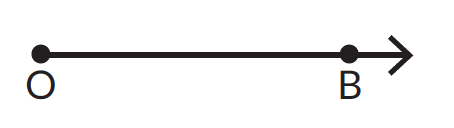## Tips and Tricks

• Tip - Children are used to naming any straight path as a line. Help them distinguish between the lines, line segments and rays by observing the way it has been represented. A line always has two arrows (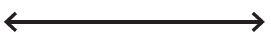) indicating that it can be extended infinitely in both directions. A line segment has two dots () indicating the end points. A ray has one dot and an arrow (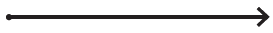) showing a definite start point and an indefinite end point.
• Keep in mind how a line, line segment and ray is denoted in letters. It will help you understand faster.

### Common mistakes or misconceptions

Misconception: In the image given below AC is the line segment.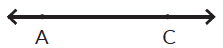A line segment is denoted by two end points showing the definite length. A line is always denoted by two arrows showing its indefinite length. Therefore, AC is a line.

Misconception: In the image given below ray OA can also be written as ray AO.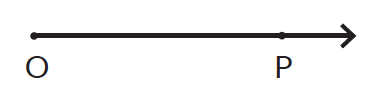Ray OP cannot be written as ray PO because ‘O’ is the point of origin extending indefinitely towards P.

Misconception: A line can be measured.When the two end points on the line are defined only then it can be measured. In the image above line segment AC can be measured.

## Depict the following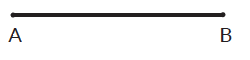1. AB is a ______________________________.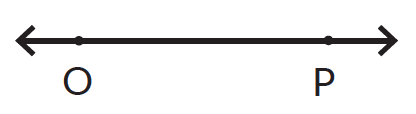2. OP is a _____________________________.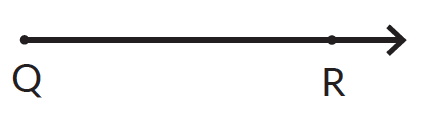3. QR is a _____________________________________.

%--split--%

## Text Solution

More Important Topics
Numbers
Algebra
Geometry
Measurement
Money
Data
Trigonometry
Calculus
More Important Topics
Numbers
Algebra
Geometry
Measurement
Money
Data
Trigonometry
Calculus